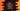# Finding out random numbers in Kotlin## Introduction :

We have a couple of different ways to generate random numbers in Java. Starting from Kotlin 1.3, one new package kotlin.random was introduced for a pseudo-random generation. So, if your project is using 1.3 or later Kotlin version, you can use this package.

In this post, I will mainly show you a couple of different methods to generate random numbers. If you get confused about which one to prefer, just use kotlin.random.

### Using java.util.Random :

java.util package provides one class called Random to create random numbers. To use this class on Kotlin, import it :

``import java.util.Random``

and, use it like below :

``print(Random().nextInt(20))``

Here, the parameter 20 is optional. It is called bound. This is the upper bound of random numbers. It will create one random number between 0(inclusive) and 20(exclusive). This value should always be positive.

Random class also provides other methods to generate random boolean, double, float, bytes and long.

### Using java.util.concurrent.ThreadLocalRandom :

ThreadLocalRandom can be used to generate positive or negative random numbers as double, integer or long. It is useful in the multithreaded environment because this is a thread-safe random number generator. It generates different Random number generator for each thread and thus provides more concurrency safety.

import it :

``import java.util.concurrent.ThreadLocalRandom``

and use it like below :

``````import java.util.concurrent.ThreadLocalRandom
fun main(args: Array<string>) {
}``````

nextInt takes two parameters. The first one is the lower bound(inclusive) and the second one is the upper bound(exclusive).

### Shuffling range values :

This is another way to generate a random number. We will shuffle one list of numbers and pick the first or the last value from the shuffled list :

``````fun main(args: Array<string>) {
println((0..50).shuffled().first())
println((0..50).shuffled().last())
}``````

It works. But an expensive operation for a large list of numbers.

### Kotlin Random class :

Kotlin Random class provides a couple of different methods to create random integer, double, long, float, boolean and bits/bytes. To use this class, you need to import :

``import kotlin.random.Random``

Use the below methods to create a random value :

``````import kotlin.random.Random
fun main(args: Array<string>) {
println(Random.nextBoolean())
println(Random.nextInt())
println(Random.nextDouble())
println(Random.nextLong())
println(Random.nextFloat())
}``````

nextBoolean: Generates one random boolean value nextInt: Generates one random integer between Int.MINVALUE_ and Int.MAXVALUE_ (inclusive). nextDouble: Generates one random double between 0 (inclusive) and 1 (exclusive). nextLong: Generates one random long between Long.MINVALUE_ and Long.MAXVALUE_ (inclusive). nextFloat: Generates one random float between 0 (inclusive) and 1 (exclusive).

It also provides methods to create random numbers in user given range :

``````import kotlin.random.Random
fun main(args: Array<string>) {
println(Random.nextInt(10,20))
println(Random.nextDouble(10.5,20.5))
println(Random.nextLong(10,100))
}``````

It generates random values in the range provided. The first argument is the inclusive lower bound and the second argument is the exclusive upper bound.

Similarly, we can also create random bits and bytes :

``````fun nextBits(bitCount: Int): Int

fun nextBytes(array: ByteArray): ByteArray
fun nextBytes(size: Int): ByteArray
fun nextBytes(array: ByteArray, fromIndex: Int, toIndex: Int): ByteArray``````

### Kotlin random with ranges :

Kotlin Ranges comes with a method called random() to get one random value :

``````fun main(args: Array<string>) {
println((1..100).random())
println(('a'..'z').random())
}``````

This is more preferred than the shuffled method we have seen above.

### Using seed :

We can create one instance of a random number generator with an integer or long value as its seed. The generator object depends on the seed we provide. If we use the same seed for two instances, both instances will generate the same sequence of random values in the same version of Kotlin runtime.

``````import kotlin.random.Random
fun main(args: Array<string>) {
println(Random(20).nextInt())
println(Random(20).nextInt())
}``````

Here, both will print the same result as the seed is the same for both instances.